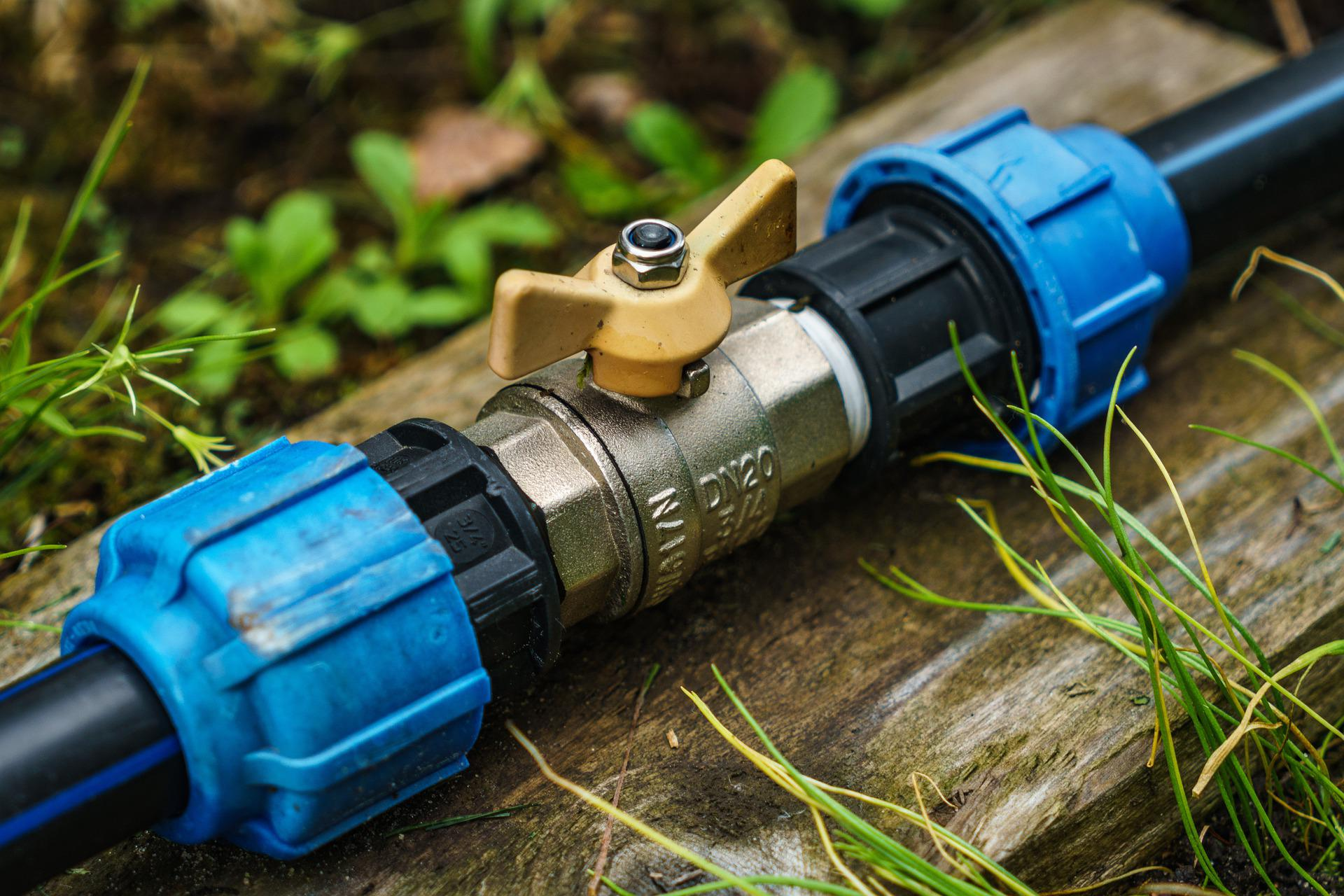## How to Check if a Horizontal Pipe Carrying Liquid is Full

In this post I want to share how to check if a horizontal pipe carrying liquid is full or partially full. We can use the following criteria to check:

• The pipe is full if: Q/d5 ≥ 10.2
• The pipe is partially full if: Q/d5 < 10.2

For partially full pipe, we need to do partially full flow analysis as follows.

x = ln (Q/d2.5)

Then we calculate height of liquid in the pipe using the following equation:

H/D = 0.446 + 0.272x + 0.0397x2 – 0.0153x3 – 0.003575x4 Read More